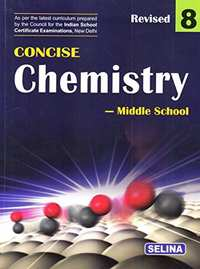Share

# Selina solutions for Concise Chemistry Class 8 ICSE chapter 5 - Language of Chemistry [Latest edition]

Course
Textbook page

#### Chapters## Chapter 5: Language of Chemistry

Exercise

### Selina solutions for Concise Chemistry Class 8 ICSE Chapter 5 Language of Chemistry Exercise [Page 67]

Exercise | Q 1.1 | Page 67

Exercise | Q 1.2 | Page 67

Define of Valency.

Exercise | Q 1.3 | Page 67

Define of Molecular formula.

Exercise | Q 2 | Page 67

Give the symbols and valencies of following radicals:
(a) Hydroxide (b) Chloride
(c) Carbonate (d) ammonium
(e) Nitrate

Exercise | Q 3.1 | Page 67

Write the molecular formula for the oxide and sulphide of following elements of Sodium.

Exercise | Q 3.2 | Page 67

Write the molecular formula for the oxide and sulphide of following elements of Calcium

Exercise | Q 3.3 | Page 67

Write the molecular formula for the oxide and sulphide of following elements of Hydrogen

Exercise | Q 4.1 | Page 67

Write the molecular formulae for the following compounds and name the elements present of  Baking soda.

Exercise | Q 4.2 | Page 67

Write the molecular formulae for the following compounds and name the elements present of  Common salt.

Exercise | Q 4.3 | Page 67

Write the molecular formulae for the following compounds and name the elements present of Sulphuric acid

Exercise | Q 4.4 | Page 67

Write the molecular formulae for the following compounds and name the elements present of Nitric acid.

Exercise | Q 5.1 | Page 67

The valency of aluminium is 3. Write the valency of other radicals present in the following compounds of Aluminium chloride.

Exercise | Q 5.2 | Page 67

The valency of aluminium is 3. Write the valency of other radicals present in the following compounds of Aluminium oxide.

Exercise | Q 5.3 | Page 67

The valency of aluminium is 3. Write the valency of other radicals present in the following compounds of Aluminium nitride

Exercise | Q 5.4 | Page 67

The valency of aluminium is 3. Write the valency of other radicals present in the following compounds of Aluminium sulphate.

Exercise | Q 6 | Page 67

What is variable valency ? Give two examples of elements showing variable valency.

Exercise | Q 7.1 | Page 67

What is a chemical equation ?

Exercise | Q 7.2 | Page 67

Why it is necessary to balance a chemical equation ?

Exercise | Q 7.3 | Page 67

What are the limitations of a chemical equation ?

Exercise | Q 8 | Page 67

Exercise | Q 9 | Page 67

State the law of conservation of mass.

Exercise | Q 10.1 | Page 67

Differentiate between:

Reactants and products.

Exercise | Q 10.2 | Page 67

Differentiate between:

A balanced and an unbalanced chemical equation

Exercise | Q 11.1 | Page 67

Balance the following equation:

"N"_2 +"H"_2→ "NH"_3

Exercise | Q 11.2 | Page 67

Balance the following equation:

"H"_2+"O"_2→"H"_2"O"

Exercise | Q 11.3 | Page 67

Balance the following equation:

"Na"_2"O"+"H"_2"O"→ "NaOH"

Exercise | Q 11.4 | Page 67

Balance the following equation:

"CO"+"O"_2→"CO"_2

Exercise | Q 11.5 | Page 67

Balance the following equation:

"Zn"+"HCI"→ "ZnCI"_2+"H"_2

Exercise | Q 12.1 | Page 67

Write a balanced chemical equation for the following word equation:

Iron + Chlorine → Iron (III) chloride

Exercise | Q 12.2 | Page 67

Write balanced chemical equations for the following word equation:

Magnesium + dil sulphuric acid → Magnesium sulphate + hydrogen gas

Exercise | Q 12.3 | Page 67

Write balanced chemical equations for the following word equation:

Magnesium + oxygen → Magnesium oxide

Exercise | Q 12.4 | Page 67

Write balanced chemical equations for the following word equation:

Calcium oxide + water → Calcium hydroxide

Exercise | Q 12.5 | Page 67

Write balanced chemical equations for the following word equation:

Sodium + chlorine → Sodium chloride

Exercise | Q 13 | Page 67

What information do you get from the following chemical equation:
Zn(s) + 2HCl (dil) → ZnCl2 (aq) + H2(g)

## Chapter 5: Language of Chemistry

Exercise## Selina solutions for Concise Chemistry Class 8 ICSE chapter 5 - Language of Chemistry

Selina solutions for Concise Chemistry Class 8 ICSE chapter 5 (Language of Chemistry) include all questions with solution and detail explanation. This will clear students doubts about any question and improve application skills while preparing for board exams. The detailed, step-by-step solutions will help you understand the concepts better and clear your confusions, if any. Shaalaa.com has the CISCE Concise Chemistry Class 8 ICSE solutions in a manner that help students grasp basic concepts better and faster.

Further, we at Shaalaa.com provide such solutions so that students can prepare for written exams. Selina textbook solutions can be a core help for self-study and acts as a perfect self-help guidance for students.

Concepts covered in Concise Chemistry Class 8 ICSE chapter 5 Language of Chemistry are Symbols of Elements, Formulae of Compounds, Balancing Simple Equations, Relate the Law to the Balancing of Simple Equations, Information Gathered from a Chemical Equation, Limitations of a Chemical Equation:Catalyst, Conditions, Language of Chemistry - Law of Conservation of Mass, Concept for Language of Chemistry.

Using Selina Class 8 solutions Language of Chemistry exercise by students are an easy way to prepare for the exams, as they involve solutions arranged chapter-wise also page wise. The questions involved in Selina Solutions are important questions that can be asked in the final exam. Maximum students of CISCE Class 8 prefer Selina Textbook Solutions to score more in exam.

Get the free view of chapter 5 Language of Chemistry Class 8 extra questions for Concise Chemistry Class 8 ICSE and can use Shaalaa.com to keep it handy for your exam preparation